Test: Diode Circuit

# Test: Diode Circuit

Test Description

## 30 Questions MCQ Test Power Electronics | Test: Diode Circuit

Test: Diode Circuit for Electrical Engineering (EE) 2023 is part of Power Electronics preparation. The Test: Diode Circuit questions and answers have been prepared according to the Electrical Engineering (EE) exam syllabus.The Test: Diode Circuit MCQs are made for Electrical Engineering (EE) 2023 Exam. Find important definitions, questions, notes, meanings, examples, exercises, MCQs and online tests for Test: Diode Circuit below.
Solutions of Test: Diode Circuit questions in English are available as part of our Power Electronics for Electrical Engineering (EE) & Test: Diode Circuit solutions in Hindi for Power Electronics course. Download more important topics, notes, lectures and mock test series for Electrical Engineering (EE) Exam by signing up for free. Attempt Test: Diode Circuit | 30 questions in 30 minutes | Mock test for Electrical Engineering (EE) preparation | Free important questions MCQ to study Power Electronics for Electrical Engineering (EE) Exam | Download free PDF with solutions
 1 Crore+ students have signed up on EduRev. Have you?
Test: Diode Circuit - Question 1

### An ideal diode has _________ &__________

Detailed Solution for Test: Diode Circuit - Question 1

An ideal diode has no losses and negligible reverse recovery time.

Test: Diode Circuit - Question 2

### A diode circuit is so arranged that when the switch is open it’s KVL gives Ri+ 1/C ∫i dt = 0 When the switch is closed, Ri+ 1/C ∫i dt = Vs Vs is the dc supply voltage. The diode is so connected that it is forward biased when switch is closed The circuit is mostly likely be a

Detailed Solution for Test: Diode Circuit - Question 2

Examine the equation, the same current flows through R & C. Also when switch is open, the equation R.H.S is 0. Hence, all the elements are in series.

Test: Diode Circuit - Question 3

### A circuit is so formed such that the source-R-C-diode-switch are in series. Consider the initial voltage across the C to be zero. The diode is so connected that it is forward biased when the switch is closed. When the switch is closed,

Detailed Solution for Test: Diode Circuit - Question 3

Instant switch is closed, the current is maximum Vs/R than starts to reduce, whereas voltage starts to increase from 0 to Vs (Capacitor Charging).

Test: Diode Circuit - Question 4

The time constant of a series RC circuit (τ) is given by

Detailed Solution for Test: Diode Circuit - Question 4

For a series RC circuit, τ = RC.

Test: Diode Circuit - Question 5

A circuit is so formed such that source-R-L-diode-switch are all in series. Consider the initial current in L to be zero. The diode is so connected that it is forward biased when switch is closed.
When the switch is closed,

Detailed Solution for Test: Diode Circuit - Question 5

Instant switch is closed, the current is minimum zero than starts to increase till it reaches a constant value Vs/R, whereas voltage starts to reduce from Vs to 0 (Inductor Charging).

Test: Diode Circuit - Question 6

In the figure shown below,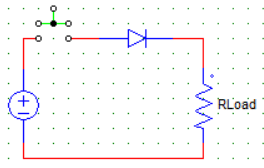As the switch (shown in the green) is pressed, the voltage across the diode(ideally)

Detailed Solution for Test: Diode Circuit - Question 6

As the switch is pressed, current starts to flow & the whole supply voltage (ideally) appears across the load R & voltage across the diode is zero.

Test: Diode Circuit - Question 7

For a diode circuit the voltage across the capacitor is given by
Vc(t)= Vs(1-e(-t/RC))
Then the initial rate of change of capacitor voltage is given by

Detailed Solution for Test: Diode Circuit - Question 7

Find d(Vc)/dt and put t = 0.

Test: Diode Circuit - Question 8

In the circuit show below,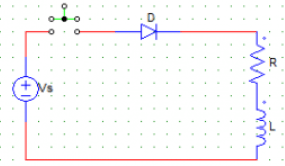The initial current through the inductor is zero. When the switch (shown in green) is closed, then the current through the inductor

Detailed Solution for Test: Diode Circuit - Question 8

Current increases from zero to maximum value gradually due to the L nature. The KVL when switch is closed gives,
Ri + Ldi/dt = Vs
Solve for i(t). Maximum value comes out to be Vs/R.

Test: Diode Circuit - Question 9

In the figure shown below,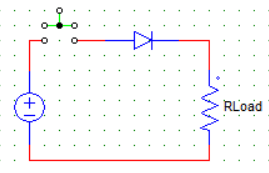When the switch is open, the voltage across the diode (ideally)

Detailed Solution for Test: Diode Circuit - Question 9

When the switch is open, the diode experiences all the supply voltage.

Test: Diode Circuit - Question 10

The time constant of a series RL circuit (τ) is given by​

Detailed Solution for Test: Diode Circuit - Question 10

The time constant τ for a series RL circuit is R/L.

Test: Diode Circuit - Question 11

For the initially relaxed circuit shown below, KVL with switch in the closed position gives a certain equation. The Laplace of this equation will have the right hand side (RHS) as​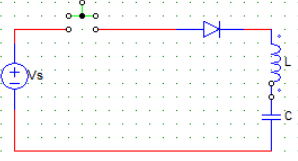Detailed Solution for Test: Diode Circuit - Question 11

When switch is closed,
Ldi/dt + 1/C ∫ idt = Vs
Laplace of the above gives,
L[sI(s)] + 1/C [I(s)/s] = Vs/s.

Test: Diode Circuit - Question 12

For the initially relaxed circuit shown below, the Laplace transform of the KVL when the switch is closed is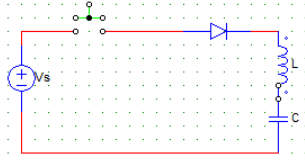I(s) [ X ] = Vs/s
The value of X is

Detailed Solution for Test: Diode Circuit - Question 12

When switch is closed,
Ldi/dt + 1/C ∫ idt = Vs
Laplace of the above gives,
L[sI(s)] + 1/C [I(s)/s] = Vs/s.

Test: Diode Circuit - Question 13

For the initially relaxed circuit shown below, if Ω=1/√LC. Then the current is a function of ___​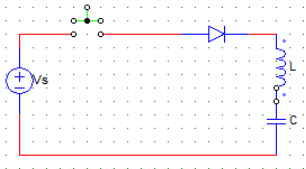Detailed Solution for Test: Diode Circuit - Question 13

When switch is closed,
Ldi/dt + 1/C ∫ idt = Vs
Laplace of the above gives,
L[sI(s)] + 1/C [I(s)/s] = Vs/s
I(s) = Vs/(LΩ) * (Ω/Ω2 + s2)
Taking the inverse lapace gives,
I(t) = Vs * √ (L/C) * sin Ωt.

Test: Diode Circuit - Question 14

For the circuit shown below, the capacitor is initially charged to a voltage of Vo with the upper plate positive. After the switch (shown in green) is closed, the current through the load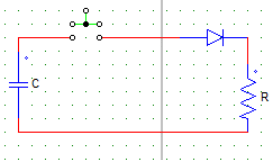Detailed Solution for Test: Diode Circuit - Question 14

The capacitor acts as a source. At instant switch is closed the current is maximum and than discharges till zero value through the load R.

Test: Diode Circuit - Question 15

For the circuit shown below, the capacitor is initially charged to a voltage of Vo with the upper plate positive. Switch is closed at t=0. The peak value of the current through the diode is​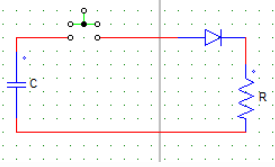Detailed Solution for Test: Diode Circuit - Question 15

When switch is closed, the equation is
Ri + 1/C ∫idt = 0 (Voltage across capacitor cannot change instantaneously)
Solution of the above equation gives, Vo/R at t= 0.

Test: Diode Circuit - Question 16

When the switch (shown in green) is closed, the average current through the diode in the positive cycle is​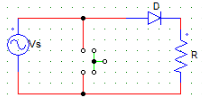Detailed Solution for Test: Diode Circuit - Question 16

The switch S.C’s the source.

Test: Diode Circuit - Question 17

When the switch is closed, the steady state current through the diode is​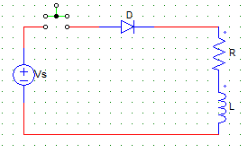Detailed Solution for Test: Diode Circuit - Question 17

I = Vo/R

Test: Diode Circuit - Question 18

When the switch is open, the current through the diode in the positive cycle is​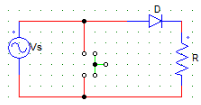Detailed Solution for Test: Diode Circuit - Question 18

When the switch is open, the diode is forward biased and I = Vs/(Rd + R). Where, Rd is the diode resistance.

Test: Diode Circuit - Question 19

For the circuit shown in the figure below, consider the diode as an ideal diode & R.M.S value of source voltage as Vs.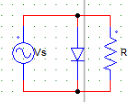The output voltage waveform at R is most likely to have

Detailed Solution for Test: Diode Circuit - Question 19

The diode S.C’s the load in the positive half cycle.

Test: Diode Circuit - Question 20

For the circuit shown in the figure below, V8 is AC voltage source with peak value Vm. The waveform of the load voltage at the resistor is​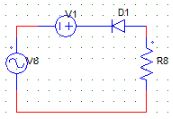Detailed Solution for Test: Diode Circuit - Question 20

Diode is reversed biased in the positive half cycle. In the negative half cycle, apply KVL to get the value of peak voltage at the load.

Test: Diode Circuit - Question 21

For the circuit shown in the figure below, consider the diode as an ideal diode & rms value of source voltage as Vs.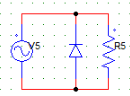The output voltage waveform at R will have

Detailed Solution for Test: Diode Circuit - Question 21

The diode short circuits the load in the negative half cycle. The peak value in the positive half is 1.414 x Vs.

Test: Diode Circuit - Question 22

For the circuit shown in the figure below, Vs is the ac voltage source with peak value Vm. The waveform of the load voltage at the resistor will have​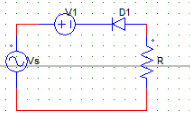Detailed Solution for Test: Diode Circuit - Question 22

Diode is reversed biased in the positive half cycle. In the negative half cycle, apply KVL to get the value of peak voltage at the load.

Test: Diode Circuit - Question 23

For the circuit shown below,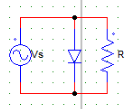Vs=230V, Voltage drop across the diode (Vd) = 2V
The peak value of voltage at R in the positive and the negative half cycles are ___ & ___ respectively.

Detailed Solution for Test: Diode Circuit - Question 23

Load is S.C in the positive half cycle hence, voltage is zero. In negative half the peak value is (230 x 1.414) – 2(drop across the diode) = 323 Volts.

Test: Diode Circuit - Question 24

For the circuit shown in the figure below, V9 is the AC voltage source with peak value Vm. The waveform of the load voltage at the resistor has a​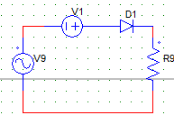Detailed Solution for Test: Diode Circuit - Question 24

Diode is reversed biased in the negative half cycle. In the positive half cycle, apply KVL to get the value of peak voltage at the load. Peak value = Vm + V1, as V1 is aiding the positive anode of the diode.

Test: Diode Circuit - Question 25

The circuit shown below has the following parameters: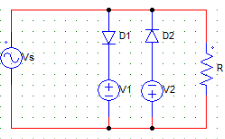V1 = 8 Volts
V2 = 6 Volts
Vs = 10V/√2 (rms)
Voltage drop across D1 & D2 = 0.7 Volts
At the load (R), the peak value in the positive half cycle will be

Detailed Solution for Test: Diode Circuit - Question 25

In the positive half, only D1 is active. Hence use KVL, Vo = 8 + 0.7 Volts.

Test: Diode Circuit - Question 26

The circuit shown below has the following parameters: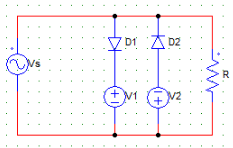Voltage drop across D1 & D2 = 0.7 Volts
V1 = 8 Volts
V2 = 6 Volts
Vs = 10V/√2 (RMS)
At the load (R), the peak value in the negative half cycle is

Detailed Solution for Test: Diode Circuit - Question 26

In the negative half, only D2 is active. Hence use KVL, Vo = 6 + 0.7 Volts.

Test: Diode Circuit - Question 27

Consider the diode to be an ideal one and Vo = Vr + Vdc during positive half cycle. Thus, during the negative half cycle​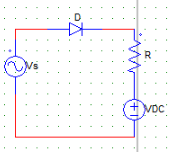Detailed Solution for Test: Diode Circuit - Question 27

During the negative half diode is not conducting hence Vr (voltage across the resistor) = 0. Vo = Vdc.

Test: Diode Circuit - Question 28

When a diode is connected in series with an AC source & R load, the conduction time per cycle is

Detailed Solution for Test: Diode Circuit - Question 28

The diode is forward biased for half cycle (180 degrees) and reversed biased in the another 180 degrees. Hence, per cycle it only conducts for 180 degrees or π radians.

Test: Diode Circuit - Question 29

When diode is connected in series to an AC source & RL load, the conduction time for the diode

Detailed Solution for Test: Diode Circuit - Question 29

For an R – L load, the inductively load can make the diode to force conducted hence, the conduction time can be greater than 180 degrees.

Test: Diode Circuit - Question 30

For the circuit shown below,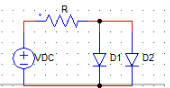Vdc = 50 V
Cut-in voltage for D1 = 0.2 V
Cut-in voltage for D2 = 0.6 V
R = 5KΩ
Current through D1 & D2 would be,

Detailed Solution for Test: Diode Circuit - Question 30

As the cut-in voltage for D1 is lesser than D2, D1 would start conducting first & S.C D2. Hence, only D1 conducts with I = 50/5000 A.

## Power Electronics

5 videos|39 docs|63 tests
Information about Test: Diode Circuit Page
In this test you can find the Exam questions for Test: Diode Circuit solved & explained in the simplest way possible. Besides giving Questions and answers for Test: Diode Circuit, EduRev gives you an ample number of Online tests for practice

## Power Electronics

5 videos|39 docs|63 tests(Scan QR code)